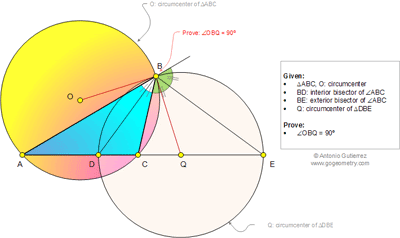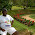## Wednesday, April 16, 2014

### Geometry Problem 1005: Triangle, Circle, Circumcenter, Interior and Exterior Angle Bisector, 90 Degree, Perpendicular

Level: Mathematics Education, High School, Honors Geometry, College.

Click the figure below to see the complete problem 1005.1.Let BD cut circle O at F
Draw altitude BH of triangle ABC
F is the midpoint of arc AC => OF ⊥AC
∠(OFB)= ∠(FBH)= ∠(BEH)
Since ∆(OBF) and ∆(BQE) are isosceles => ∠(OBF)= ∠(QBE)
So ∠(OBQ)=90 degrees

2.<DBE=90, and <EBQ=90-(<A+<B/2)=B/2+90-(<A+<B)=<ABD+<OBA=<OBD, so <OBQ=<DBE

3.Let be B,F, intersection points of circles.
FQB isoceles, QO altitude => QO bisector => QB tg to OB

4.Since QD = QB, < QDB = < QBD so < QBC = < BAC

Hence QB is a tangent to circle ABC at B and so OB has to be perpendicular to QB

Sumith Peiris
Moratuwa
Sri Lanka

5.Problem 1005
Solution 1
In triangle ABC by the theorem of the internal and external dichotomy we have
AB/BC=AD/DC=AE/EC. Is <DBE=90 so the Q is midpoint DE. From theorem
Newton apply QD^2=QC.QA=QB^2 so QB is tangent meets the circle with center O.
Solution 2
<CBQ=<CBE-<QBE=(<BAC+<ACB)/2-<QEB=(90-<ABC/2)-(90-<QDB)=<QDB-<ABC/2=<BAC+<ABC/2-<ABC/2=<BAC. So QB is tangent meets the circle with center O.
APOSTOLIS MANOLOUDIS 4 HIGH SCHOOL KORYDALLOS PIRAEUS GREECE